# Water viscosity

Jump to: navigation, search

## Water viscosity

Water viscosity correlation is published by McCain in 1991.

## Math and Physics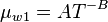$\mu_{w1} = AT^{-B}$

where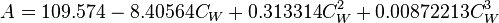$A = 109.574 - 8.40564 C_W + 0.313314 C_W^2 + 0.00872213 C_W^3$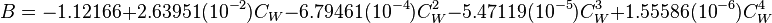$B = -1.12166 + 2.63951(10^{-2}) C_W - 6.79461(10^{-4}) C_W^2 - 5.47119(10^{-5}) C_W^3 + 1.55586(10^{-6}) C_W^4$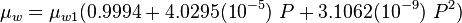$\mu_{w} = \mu_{w1} (0.9994 + 4.0295(10^{-5})\ P + 3.1062(10^{-9})\ P^2)$

## Example. Calculating water viscosity

### Input data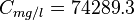$C_{mg/l} = 74289.3$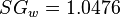$SG_w = 1.0476$

Calculate water viscosity at 3176 psia and 165°F?

### Solution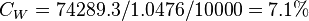$C_W = 74289.3 / 1.0476 / 10000 = 7.1%$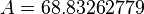$A=68.83262779$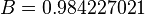$B=0.984227021$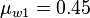$\mu_{w1} = 0.45$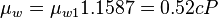$\mu_w = \mu_{w1} 1.1587 = 0.52 cP$

The solution is also available in the PVT software model at [The solution is available in the PVT calculator software model at www.pengtools.com

## Nomenclature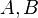$A, B$ = coefficients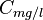$C_{mg/l}$ = pressure correction, res bbl/STB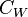$C_W$ = water salinity or weight percent solids, %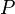$P$ = pressure, psia$T$ = Temperature, °F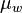$\mu_w$ = water viscosity, cP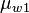$\mu_{w1}$ = water viscosity at 1 atm, cP

## See also

Water bubble point pressure
Water compressibility
Water density
Water formation volume factor
Water salinity from density equation
Water solids concentration
Water viscosity
Gas/Water Interfacial Tension